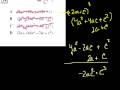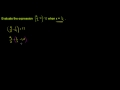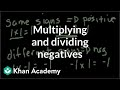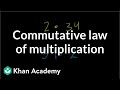# Multiplication Videos

## CA Standards: Algebra IISal from Khan Academy works through polynomial factoring and multiplication problems that involve geometric images. A [...]

## Associative Property for MultiplicationSal from Khan Academy explains the concept behind the associative proper for multiplication. Learn the definition [...]

## Multiplying and Dividing Negative NumbersSal from Khan Acadmey makes multiplying and dividing negative numbers simple by explaining the basic rules [...]

## Solving Systems of Equations by MultiplicationSal from Khan Academy explains how to solve systems of equations by multiplication. Using the simple [...]

## Multiplication of Rational NumbersSal fom Khan Academy takes you through several examples of multiplying rational numbers. After multiplying these [...]

## Commutative Law of MultiplicationSal from Khan Academy explains the principles behind the commutative law of multiplication. The concept sounds [...]

## More on Matrix Addition and Scalar MultiplicationSal from Khan Academy further explains matrix addition and scalar multiplication. A complicated topic becomes easier [...]

## Multiplication of PolynomialsSal from Khan Academy demonstrates how to multiply polynomials. He gives tons of examples, and with [...]

## Multiplication 3: 10,11,12 times tablesSal from Khan Academy goes over the multiplication tables for numbers 10, 11, and 12. He [...]

## Multiplication 4: 2-digit times 1-digit numberAre you ready to move on to more difficult multiplication problems? Sal from Khan Academy demonstrates [...]